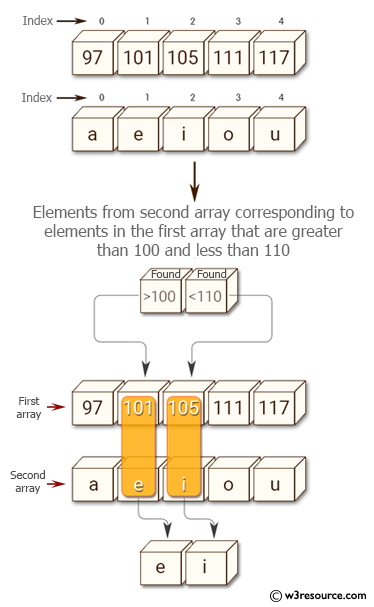﻿ NumPy: Select indices satisfying multiple conditions in a NumPy array - w3resource# NumPy: Select indices satisfying multiple conditions in a NumPy array

## NumPy: Array Object Exercise-92 with Solution

Write a NumPy program to select indices satisfying multiple conditions in a NumPy array.
Sample array:
a = np.array([97, 101, 105, 111, 117])
b = np.array(['a','e','i','o','u'])

Note: Select the elements from the second array corresponding to elements in the first array that are greater than 100 and less than 110

Pictorial Presentation:Sample Solution:

Python Code:

``````import numpy as np
a = np.array([97, 101, 105, 111, 117])
b = np.array(['a','e','i','o','u'])
print("Original arrays")
print(a)
print(b)
print("Elements from the second array  corresponding to elements in the first array  that are greater than 100 and less than 110:")
print(b[(100 < a) & (a < 110)])
```
```

Sample Output:

```Original arrays
[ 97 101 105 111 117]
['a' 'e' 'i' 'o' 'u']
Elements from the second array  corresponding to elements in the first
array  that are greater than 100 and less than 110:
['e' 'i']
```

Python Code Editor:

Have another way to solve this solution? Contribute your code (and comments) through Disqus.

What is the difficulty level of this exercise?

Test your Python skills with w3resource's quiz

﻿

## Python: Tips of the Day

List comprehension:

```>>> m = [x ** 2 for x in range(5)]
>>> m
[0, 1, 4, 9, 16]
```